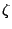# Equivalences involving primes

The main point of [Riemann's original paper] of 1859 is that the two sequences, of prime numbers on the one hand, and of zeros ofon the other hand, are in duality. A precise mathematical formulation of this fact is given by the so-called explicit formulas of prime number theory (Riemann, von Mangoldt, Guinand, Weil). Therefore, any statement about one of these two sequences must have a translation in terms of the other. This is the case for the Riemann hypothesis.

Back to the main index for The Riemann Hypothesis.